407 493 6601

# 7th Grade - Linear Inequalities in One Variable

## Introduction

• An inequality is a mathematical sentence that makes a non-equal comparison between two mathematical expressions.
• There are four inequality operators, as shown in the table below:
 Operator Symbol Less than < Graeter than > Less than or equal to $\le$ Greater than or equal to $\ge$
• Examples of inequality include: $2x>4$$5y<20$$4m-2\ge 6$, etc.

## Solving simple linear inequalities

• Inequalities can be solved using the rules mentioned below.
• Adding the same number to each side of an inequality produces an equivalent inequality wherein the sign of inequality remains unaffected.
• Subtraction Rule:
• Subtracting the same number from both sides of an inequality results in an equivalent inequality wherein the sign of inequality remains unaffected.
• Multiplication Rule:
• Multiplying both sides of an inequality by a positive number results in an equivalent inequality wherein the sign of inequality remains unaffected.
• Multiplying both sides of an inequality by a negative number results in a new inequality wherein the sign of inequality is reversed.
• Division Rule:
• Dividing both sides of an inequality by a positive number results in an equivalent inequality wherein the sign of inequality remains unaffected.
• Dividing both sides of an inequality by a negative number results in a new inequality wherein the sign of inequality is reversed.

## Graphical Representation of Linear Inequalities in One Variable

• Linear inequalities in one variable can be visualized on a number line.
• Plotting linear inequalities in one variable involves the following steps:
• First, solve the linear inequality to find the variable's value that satisfies the inequality.
• Locate and mark the solution on the number line.
• We use an open dot for strict inequalities to indicate that the point is not part of the solution.
• We use a closed dot for slack inequalities to indicate that the point is part of the solution.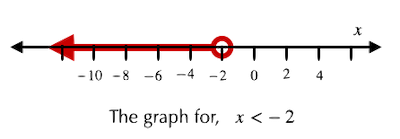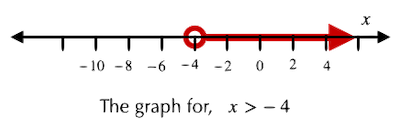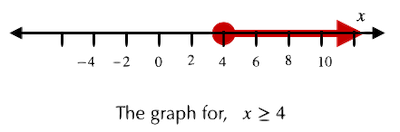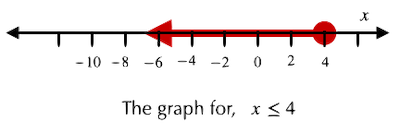## Solved Examples

Question 1: Solve the following inequality.

$m-2\le 5$

Solution: $m-2\le 5$

$m-2+2\le 5+2$

$m\le 7$

Question 2: Solve the following inequality.

$x+3>1$

Solution: $x+3>1$

Applying subtraction rule

$x+3-3>1-3$

$x>-2$

Question 3: Solve the following inequality.

$\frac{x}{2}\ge 4$

Solution: $\frac{x}{2}\ge 4$

Apply multiplication rule (by a positive number)

$\frac{x}{2}×2\ge 4×2$

$x\ge 8$

Question 4: Solve the following inequality.

$-\frac{m}{4}\le 3$

Solution: $-\frac{m}{4}\le 3$

Apply multiplication (by a negative number) rule

$-\frac{m}{4}×\left(-4\right)\ge 3×\left(-4\right)$ [Note that the inequality sign reversed due to multiplication by a negative number]

$m\ge -12$

Question 5: Solve the following equation.

$7x<21$

Solution: $7x<21$

Apply the division rule (by a positive number)

$\frac{7x}{7}<\frac{21}{7}$

$x<3$

Question 6: Solve the following inequality.

$2x\ge 8$

Solution: $2x\ge 8$

Apply division (by a negative number) rule

$\frac{2x}{2}\ge \frac{8}{2}$

$x\ge 4$

Question 7: What inequality does the number line shown below represents?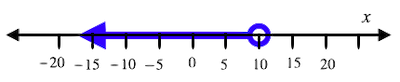Solution: An open circle is used to signify "less than (<)" or "greater than (>)" type inequality on the number line. The given figure represents $x<10$.

Question 8: What inequality does the number line shown below represents?Solution: A closed circle denotes "less than equal to ($\le$)" or "more than equal to ($\ge$)" type inequality on the number line. The given figure represents $x\ge -1$.

## Cheat Sheet

• If two mathematical expressions are separated by symbols such as >, <, $\ge$$\le$, it represents an inequality.
• To solve an inequality, we can apply the rules of addition, subtraction, multiplication, and division.
• Adding the same quantity to both sides of an inequality doesn't change the direction of the inequality sign.
• Likewise, subtracting the same quantity from both sides of an inequality doesn't change the direction of the inequality sign.
• Multiplying both sides of an inequality by a positive number leaves the inequality sign unchanged. In contrast, multiplication by a negative number reverses the direction of inequality.
• Similarly, dividing both sides of an inequality by a positive number leaves the inequality sign unchanged. At the same time, division by a negative number reverses the direction of the inequality.
• Linear inequality in one variable is plotted on a number line. An open circle is used to signify "less than (<)" or "greater than (>)", while a closed circle denotes "less than equal to ($\le$)" or "more than equal to ($\ge$)" type inequality on the number line.

## Blunder Areas

• When solving inequalities, one must not forget to reverse the inequality sign when multiplying or dividing both sides by negative numbers.